This tutorial will introduce you to the concept of a range in Google Sheets. You'll learn about what ranges are and how to use them. You'll also learn about named ranges and the benefits of using them.

A range represents a single cell or a group of adjacent cells in your spreadsheet. Every time you work with data in a spreadsheet, you're likely using one or more ranges.

The screenshot below shows 5 different ranges in Sheet3 of your spreadsheet.To reference a single cell in a formula, use the name of the sheet followed by an exclamation mark, the column and finally the row.

A cell that is in Sheet1 at the intersection of column C and row 5 will have the following reference:

. This type of reference is known as A1 notation.To reference a range composed of a group of adjacent cells, we'll need to specify the two cells that are at corners of any diagonal within the range. Typically, the cells that are at the top left and bottom right corner are the ones that are specified.

🛈 If the group of cells is fully contained within a single row or column then the top left and bottom right cells are just the first and last cells in the group.

The screenshot below displays multiple ranges (the ones that have been colored) and in each case the top left and bottom right cells have been filled with a darker color.To reference a group of cells in a formula, use the name of the sheet followed by an exclamation mark, the column of the top left cell, its row, a colon, the row of the bottom right cell and finally its column.

For example, the below references correspond to the ranges highlighted in the above screenshot.

• Range colored green:

• Range colored blue:

• Range colored: purple:

• Range colored orange:

You can also define ranges that reference entire rows or columns:

• All rows in one column: (use the column name twice and omit the row numbers)

• All rows in multiple adjacent columns: (use the names of the first and last column in the range and omit the row numbers)

• All columns in a single row: (use the row number twice and omit the column names)

• All columns in multiple adjacent rows: (use the numbers of the first and last row in the range and omit the column names)

To use a range in a function, just use the range's reference. For example, in order to calculate the sum of values in the range , use the formula . To sum values in just a single cell, say , use .In Google Sheets, you can assign a name to a range. Once you do this, you can use the name of a range instead of its reference in formulas and scripts.

There are several ways to create a named range:

1. Select Data —> Named ranges and enter the name and reference.

2. Select a range in the spreadsheet, right click and select Define named range to give it a name.

3. Create a named range by using Google Apps Script.

You can also create named ranges using Google Apps Script. The code below shows you an example of how to do that.

function createNamedRange(name, range) { SpreadsheetApp.getActive().setNamedRange(name, range); }

Once you create a named range, you can use it in formulas and scripts by using its name. So, instead of , you can use the range's name like this: .

Using named ranges has several benefits:

• Formulas and scripts will become more readable because the name of the range will help users understand the type of data contained in it. For e.g., is a lot more descriptive than .

• When you make changes to a named range, all the formulas and scripts will immediately begin using the updated range.

This tutorial covered the basics of working with ranges in Google Sheets. You learned how to:

Have feedback for me?

I'd appreciate any feedback you can give me regarding this post.

Was it useful? Are there any errors or was something confusing? Would you like me to write a post about a related topic? Any other feedback is also welcome. Thank you so much!

I’d always believed that Named Ranges in Google Sheets would only accept static ranges and not formulas like Excel, which makes them less powerful of course. (Check out the Named ranges sidebar and you’ll see what I mean. No place to add formula-based dynamic ranges in there.)

However, there is a clever trick using the INDIRECT function that DOES allow dynamic named ranges!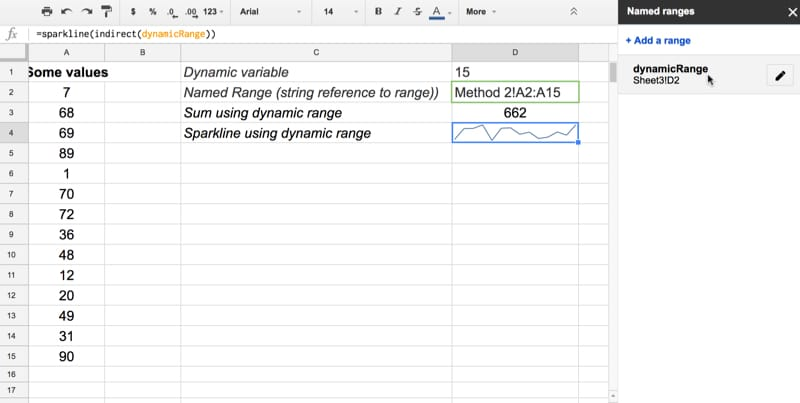### Using formulas in named ranges to make them dynamic

Step 1: Create a formula whose output is a range reference in string format, for example:

where cell B1 contains an integer that we control (typed or from a calculation).

So if cell B1 contains the integer 500 say, the output of this formula would be a text string as follows:

Step 2: Create a named range for this cell with the string in.

Click on the formula cell, click on and give it a name, e.g.

Step 3: Combine this named range with the INDIRECT function to refer to this string range inside your other formulas, which gives you a dynamic named range.

For example, to create a dynamic sum we could do this:

Or a dynamic sparkline:

Once these are setup, if you go back and change the value in cell B1, the range will update and the formulas in Step 3 will also update.

### Can I see an example worksheet?

Yes, here you go.

### How does the formula for dynamic named ranges work?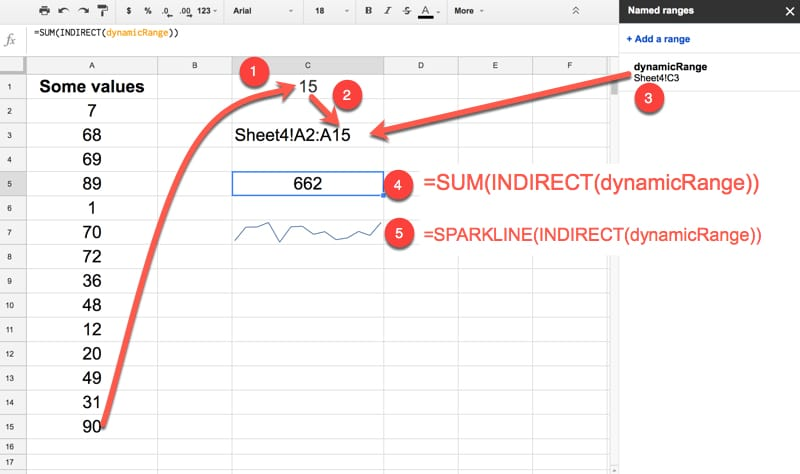1. Formula to count last row
2. Formula to create a string range reference
3. This cell is a named range: dynamicRange
4. SUM formula using INDIRECT and the named range
5. SPARKLINE formula using INDIRECT and the named range

Dynamic named ranges! Woohoo! This is one of my all time favorite Google Sheet tricks.

Sours: https://www.benlcollins.com/formula-examples/dynamic-named-ranges/

When handling large amounts of data, being able to group certain values together can be very useful. Calculating hundreds of values automatically is one of the reasons that spreadsheets were made, after all. This is where being able to declare cell ranges is important, as it simplifies what would be otherwise cumbersome computations.### How to Find the Range in Google Sheets

The definition of a range in spreadsheets is quite different from its equivalent in math. Simply put, when working in spreadsheet programs, a range is a group of selected cells. This is important because by grouping cells together, you can use these groups as values for doing calculations. This allows a user to automatically compute formulas with a range as an argument.

Finding the range in Google Sheets is a very easy process. You just start from one end of a data set to the other. For example, a data set of ten numbers has a range either from one to ten or from ten to one. It doesn’t matter where you begin or where you end, as long as it covers the entire data set, that is your range.

If you look at the top and to the left of a Google Sheet document, you’ll notice that some letters and numbers mark them. This is how you determine the name of a particular cell in the sheet. You look at the letter from the top, then look at the number to the left. The very first cell would be A1, the cell immediately to the bottom of it would be A2, and the one to the immediate right is B2. This is how you determine the first and last value of your range.

Calculating the range would be easy if it was a single row or a column. Just use both ends of the data set that has a value then put a colon in between them. For example, in one column of data starting from A1 to A10, the range would be A1:A10 or A10:A1. It doesn’t matter if you use either end first.

It gets a little complicated when you’re working with multiple rows or columns. For this kind of data set, you need to determine two opposite corners to get your range. For example, a set of nine cells composed of three rows and three columns starting from A1 and ending at C3, the opposite corners would be A1 and C3 or A3 and C1.

It makes no difference whether you take the top leftmost and the bottom rightmost cells or the bottom leftmost and the top rightmost. As long as they’re opposite corners, you will cover the entire data set. The range would then be either A1:C3, C3:A1, A3:C1, or C1:A3. It doesn’t matter which cell you use as your first range value.

Finding the value of a range by typing in values is handy when the number of data values you have is too many to be able to select it manually. Otherwise, you can type in = in an empty cell, then click and drag your mouse over the entire data set to automatically generate a data range.

### How to Create Named Ranges in Google Sheets

Named ranges become useful when you have too many range sets to keep track of. This can also help simplify calculations, as you can use the labels themselves as arguments for formulas. What’s easier to remember? =sum(a1:a10) or =sum(daily_sales)? By using the latter, not only will you know what the range is actually for, by looking at the formula alone you can see that the result is the sum of the day’s sales.

To create a named range, do the following:2. Select the range you want to name.3. Click on Data on the top menu.4. Click on Named ranges from the dropdown list. A window will pop up on the right.5. On the first textbox, type in the name that you want.6. If you wish to change the selected range, you can change the values on the second textbox. If you have multiple sheets, you can type the sheet name followed by an exclamation mark (!) to specify which sheet you’re using. The values between colon (:) is the range.7. Once you’re finished naming, click on Done.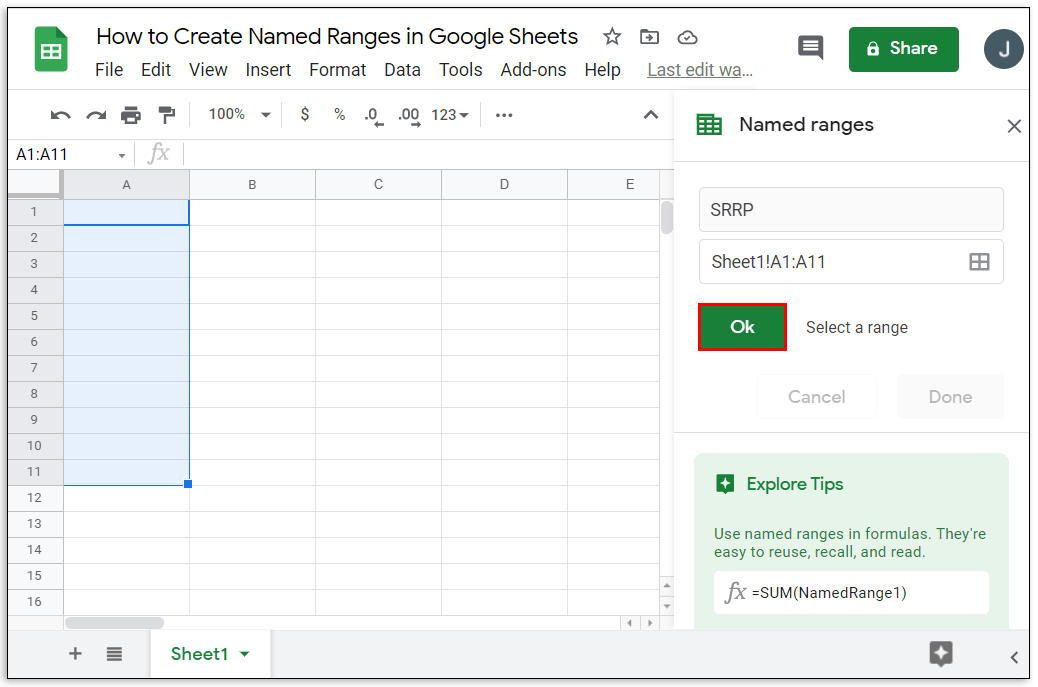There are certain rules that you must follow when naming ranges. Not adhering to these rules will often result in error messages or failure of a formula to produce a result. These rules are:

1. Range names can only contain numbers, letters, and underscores.
2. You can’t use spaces or punctuation marks.
3. Range names can’t begin with either the word true or false.
4. The name must be between one and 250 characters.

Here’s how to edit already named ranges:2. Click on Data on the top menu.3. Click on Named ranges from the dropdown menu.4. On the window to the right, click on the named range that you want to edit.5. Click on the pencil icon to the right.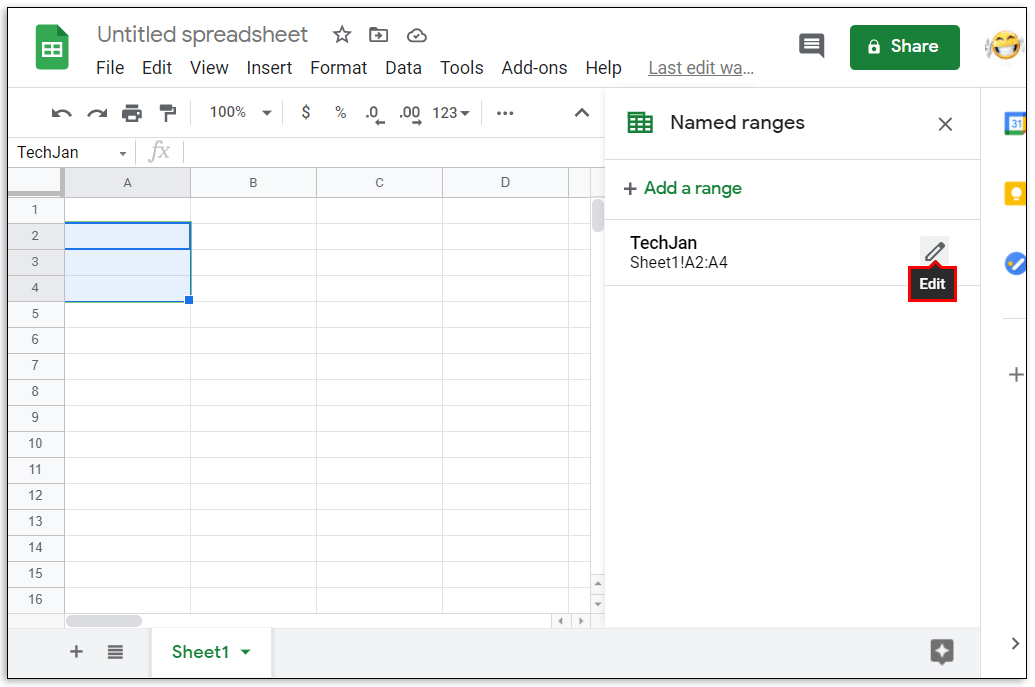6. To edit the name, type in the new name then click on Done. To delete the range name, click on the trash can icon to the right of the range name, then click on Remove on the window that pops up.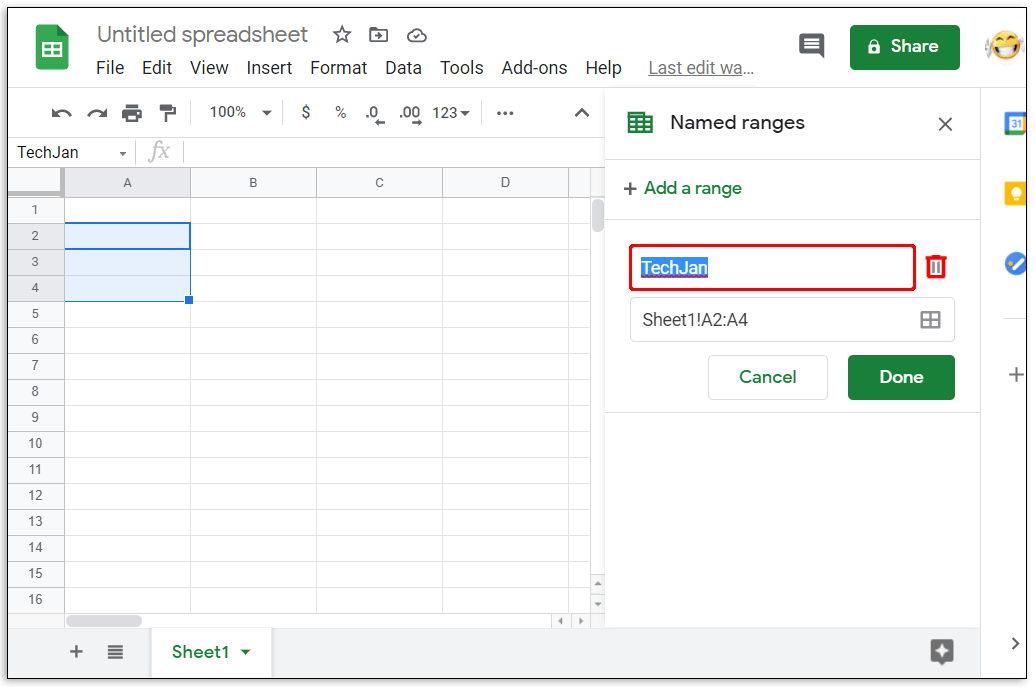### How Do You Access AVERAGE Function in Google Sheets?

If you want to use the AVERAGE function, you can do the following:

• Click on an empty cell where you wish the answer to be displayed.• On the top menu, click on Insert.• Mouse over Function on the dropdown menu.• Click on AVERAGE.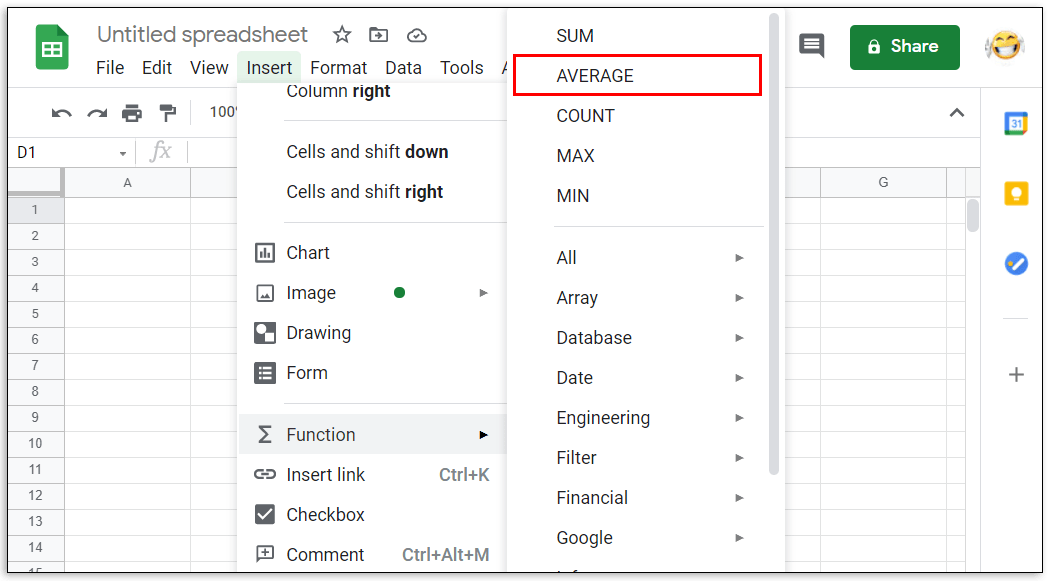• Type in the values that you want the AVERAGE function to use.• Press the enter or return key.

Changing the range is as easy as editing the first or last value of the cell numbers between the colon symbol. Remember that the range argument takes the first and last value you enter and includes all cells in between as a member of that range. Increasing or decreasing either number in between the colon will increase or decrease the members of the range accordingly.

### How Do You Calculate Total in Google Sheets?

Formulas in Google Sheets can automatically calculate the total of a certain range of cells. If the values inside the cells are changed, the total will then adjust accordingly. The usual function used is SUM which is the total of all the values in the argument. The syntax of this function is =SUM(x:y) where x and y is the start and end of your range accordingly. For example, the total of a range from A1 to C3 will be written as =SUM(A1:C3).

### How Do I Select a Data Range in Google Sheets?

You can select a range in two ways, either type in the range values manually, or click and drag your mouse over the entire range itself. Clicking and dragging is useful if the amount of data you have only spans a few pages. This becomes unwieldy if you have data that number in the thousands.

To manually select a data range, find the top leftmost value and the bottom rightmost value and place them in between a colon. The same also applies to the top rightmost and bottom leftmost values. You can then type this in as an argument in a function.

### How Do You Find the Mean in Google Sheets?

In mathematical terms, the mean is the sum of the values of a set of cells, divided by the number of cells added. Simply put, it is the average value of all the cells. This can be accomplished by using the AVERAGE function in the Insert and Function menu.

### What Is a Data Range in Google Sheets?

A data range is a set of cells that you want to use in a function or formula. It’s another name for range. The two names are interchangeable.

### What Is a Valid Range in Google Sheets?

Depending on the formula you use, some values will not be accepted as an argument. For example, the cell value TRUE can’t be used in the formula =SUM() as it’s not a calculable numeric value. A valid range is a set of cells containing data that a formula will accept as an argument. If there is a cell that has an unaccepted input, then the range isn’t valid. Invalid ranges can also occur when either the first or last point of the range has a value that results in an error.

### How Do I Find the Statistical Range of Values in Google Sheets?

In mathematics, the statistical range is the difference between the highest value and the lowest value of a set of data. Google Sheets has several functions that make the calculation of this rather simple. The MAX and MIN function is located under the Insert and Function menu. To find the statistical range or a data set just type in =(MAX(x) – MIN(x)) where x is your range. For the statistical range of a data set from A1 to A10, for example, the formula would be =(MAX(A1:A10) – MIN(A1:A10)). If you want rounded down values, you can use this syntax: =round(MAX(A1:A10),1)-round(MIN(A1:A10),1).

### Efficient Calculations

Knowing how to calculate the range in Google Sheets helps users efficiently handle vast amounts of data. You can make use of all the formulas and functions that Google Sheets has to offer more easily if you can group data in specific sets and ranges. Understanding how ranges work can help simplify your workload.

Do you know of another way on how to calculate a range in Google Sheets? Share your thoughts in the comments section below.

How to calculate range on Google Sheets

You can have cleaner formulas by using Named Ranges in Google Sheets. Let’s see how to apply the same to a range of cells and use it.

Let’s come back to the tutorial.

Here in this Google Sheets tutorial, I am covering the advantages of using named ranges in Google Sheets. In addition to this, we can learn how to name ranges in Sheets.

Ever wonder seeing an unknown function in a formula in Google Sheets?

It might be a formula that contains the so-called named range, not a function.

Please check the below table, where you can see that cells D4:D9 contains sales value. How you total it usually?

It might be as follows.

But for making your formula cleaner and user friendly, you can first name the ranges D4:D9 as “SalesValue” and use it in your formula as below.

Another advantage is you don’t need to remember the range D4:D9. Instead, memorize “SalesValue” and use it anywhere in the sheet (even in a different tab in that sheet).

### How to Name a Cell Range in Google Sheets

There are two methods that you can adopt. One is using the menu command, and another is a shortcut method.

You may be unfamiliar with the latter method as it’s relatively new.

Let’s see how to apply a name to the cell range D4:D9 using both methods.

1. Select the required cell range (here D4:D9).
2. Go to Data menu > Named ranges. It will open a sidebar panel.
3. In the given field, type an appropriate name, here “SalesValue”, for the selected range and click “Done”.

Hereafter, in formulas in that sheet, you can use “SalesValue” instead of the array D4:D9.

Example:

#### Using Shortcut Keys

Here is the quickest way to create a named range in Google Sheets.

• Here also we should first select the cell range (for example D3:D6).
• Hit the shortcut keys Ctrl + J.
• Type the required name in the “Name box”.

Note:- Instead of the keyboard shortcut, you can directly click within the “Name box” and type the required name there.

If you see the error “The name given to this range is invalid”, it may be due to the following.

### Valid Names

• A valid name must be 1 to 250 characters. It can contain letters, numbers, and _ (underscores).
• Though we can use numbers and Boolean values (TRUE or FALSE), it should not be in the beginning.
• We can’t use numbers alone.
• Must exclude spaces or punctuation.
• The name can’t be either row-column (R1C1) notation or A1 notation.

### Points to be Noted

When you insert a row or rows within the named range area, it will automatically get added to the range.

As per the above example, if I insert one new row in the range, the range reference would change from D3:D6 to D3:D7.

If you insert a row before the first row or after the last row of a named range in Google Sheets, it will not be included automatically. You must edit the named ranges to include such additional rows.

That means the named range is not fully dynamic. But there is a workaround to this problem. You can read that in detail here – Auto-Expand Named Ranges in Google Sheets to Accommodate New Rows.

For more resources, please search on this blog using the keyword “named ranges” in the search field given on the top navigation bar.

Apply named ranges in Google Sheets and make your formulas looking clean.

That’s all. Thanks for the stay. Enjoy!

Prashanth

.

Using IMPORTRANGE Function in Google Sheets

.

### You will also be interested:

.

286 287 288 289 290